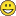# How to do a raster layer summation by loop?

774
3
11-11-2017 08:41 PMNew Contributor II

Hey folks,

I work with ArcGIS Pro and Python and I need to do a Summation over 31 raster layers. I want to do it by a loop, because I need it more often.

This is what I want to loop:

arcpy.env.workspace = Folder_Single_Layer_ND_as_0

Sum_Layer_ND_as_0 = \

Raster("/offset_0_1.tif") \

+ Raster("/offset_0_2.tif") \

+ Raster("/offset_0_3.tif") \

+ Raster("/offset_0_4.tif") \

+ Raster("/offset_0_5.tif") \

+ Raster("/offset_0_6.tif") \

+ Raster("/offset_0_7.tif") \

+ Raster("/offset_0_8.tif") \

+ Raster("/offset_0_9.tif") \

+ Raster("/offset_0_10.tif") \

+ Raster("/offset_0_11.tif") \

+ Raster("/offset_0_12.tif") \

+ Raster("/offset_0_13.tif") \

+ Raster("/offset_0_14.tif") \

+ Raster("/offset_0_15.tif") \

+ Raster("/offset_0_16.tif") \

+ Raster("/offset_0_17.tif") \

+ Raster("/offset_0_18.tif") \

+ Raster("/offset_0_19.tif") \

+ Raster("/offset_0_20.tif") \

+ Raster("/offset_0_21.tif") \

+ Raster("/offset_0_22.tif") \

+ Raster("/offset_0_23.tif") \

+ Raster("/offset_0_24.tif") \

+ Raster("/offset_0_25.tif") \

+ Raster("/offset_0_26.tif") \

+ Raster("/offset_0_27.tif") \

+ Raster("/offset_0_28.tif") \

+ Raster("/offset_0_29.tif") \

+ Raster("/offset_0_30.tif") \

+ Raster("/offset_0_31.tif") \

Sum_Layer_ND_as_0.save()

I tried so far...

# TxWx = "/offset"        # I need this as a Variable.

# Number_of_Years = 31    # I calculated this from a NetCDF

...this:

arcpy.env.workspace = Folder_Single_Layer_ND_as_0

for Number in range(1, int(Number_of_Years))

Sum_Layer_ND_as_0 += Raster(TxWx + "_0_" + Number + ".tif")

...and this:

arcpy.env.workspace = Folder_Single_Layer_ND_as_0

x = 1

while True:

Sum_Layer_ND_as_0 += Raster(TxWx + "_0_" + str(x) + ".tif")

x += int(Number_of_Years)

... and this:

arcpy.env.workspace = Folder_Single_Layer_ND_as_0

count = 1

while count <= int(Number_of_Years):

Sum_Layer_ND_as_0 += Raster(TXWX + "_0_" + str(count) + ".tif")

count +=1

Nothing works. I am a very beginner in Python. I would be very grateful if anyone could help me!!!

PS.: If my question is on the wrong position or I am not supposed to ask it here, please tell me.

Tags (5)
1 Solution

Accepted SolutionsbyMVP Legendary Contributor

You need to use list rasters and sum the raster datasets using a Local sum

``````import arcpy
from arcpy import env
env.workspace = "C:/some_folder/a_folder_or_gdb"
arcpy.CheckOutExtension("Spatial")
in_rasters = arcpy.ListRasters("*", "TIF")  # change to suit the raster types
sum_cellstats = CellStatistics_sa(in_rasters, "SUM", "DATA")  # read the help!!
sum_cellstats.save("C:/test/output/sum_o_rama")‍‍‍‍‍‍‍``````

which has obviously not been tried but you can examine the various help topics to get the idea of how to work through through the process

3 RepliesbyMVP Legendary Contributor

You need to use list rasters and sum the raster datasets using a Local sum

``````import arcpy
from arcpy import env
env.workspace = "C:/some_folder/a_folder_or_gdb"
arcpy.CheckOutExtension("Spatial")
in_rasters = arcpy.ListRasters("*", "TIF")  # change to suit the raster types
sum_cellstats = CellStatistics_sa(in_rasters, "SUM", "DATA")  # read the help!!
sum_cellstats.save("C:/test/output/sum_o_rama")‍‍‍‍‍‍‍``````

which has obviously not been tried but you can examine the various help topics to get the idea of how to work through through the processNew Contributor II

Thank you soooooo much againIt worked!byMVP Legendary Contributor

no problem